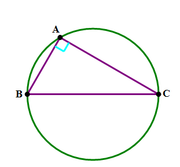## In the diagram above, what is the circumference of the

##### This topic has expert replies
Legendary Member
Posts: 2101
Joined: 29 Oct 2017
Followed by:6 members

### In the diagram above, what is the circumference of the

by swerve » Sun Jun 02, 2019 6:31 am

00:00

A

B

C

D

E

## Global StatsIn the diagram above, what is the circumference of the circle?

1) The area of triangle is $$16\sqrt{3}$$.
2) $$\angle B=60^{o}$$.

The OA is C

Source: Magoosh

### GMAT/MBA Expert

GMAT Instructor
Posts: 3008
Joined: 22 Aug 2016
Location: Grand Central / New York
Thanked: 470 times
Followed by:33 members
by [email protected] » Tue Jun 11, 2019 9:38 pm

00:00

A

B

C

D

E

## Global Stats

swerve wrote:In the diagram above, what is the circumference of the circle?

1) The area of triangle is $$16\sqrt{3}$$.
2) $$\angle B=60^{o}$$.

The OA is C

Source: Magoosh
We see that /_A = 90Âº; thus, âˆ†ABC is a right-angled triangle. And we know that only the diameter subtends an angle of 90Âº at the circumference of the circle. Thus, BC is the diameter. If we get the value of BC, we will get the value of circumference of the circle.

Let's take each statement one by one.

1) The area of triangle is $$16\sqrt{3}$$.

=> 1/2*AB*AC = 16âˆš3 => AB*AC = 32âˆš3. We can't get the value of BC. Insufficient.

2) $$\angle B=60^{o}$$.

=> âˆ†ABC is a 30-60-90 triangle. Thus, AB : AC : BC :: 1 : âˆš3 : 2. Since we do not have the actual value of any of the sides, we can't get the actual value of BC. Insufficient.

(1) and (2) together

From AB : AC : BC :: 1 : âˆš3 : 2, we have AC = âˆš3*AB and BC = 2*AB.

From AB*AC = 32âˆš3 and AC = âˆš3*AB, we have AB*AC = 32*âˆš3 = AB*(âˆš3*AB)

=> AB = âˆš32

=> BC = 2*âˆš32 = 8âˆš2.

Circumference of the circle = Ï€*BC = Ï€*8âˆš2. Sufficient.